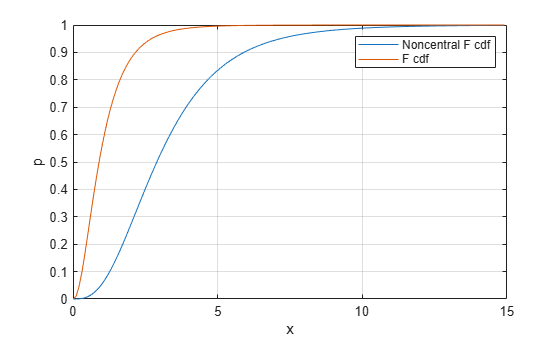# ncfcdf

Noncentral F cumulative distribution function

## Syntax

```p = ncfcdf(x,nu1,nu2,delta) p = ncfcdf(x,nu1,nu2,delta,'upper') ```

## Description

`p = ncfcdf(x,nu1,nu2,delta)` computes the noncentral F cdf at each value in `x` using the corresponding numerator degrees of freedom in `nu1`, denominator degrees of freedom in `nu2`, and positive noncentrality parameters in `delta`. `nu1`, `nu2`, and `delta` can be vectors, matrices, or multidimensional arrays that have the same size, which is also the size of `p`. A scalar input for `x`, `nu1`, `nu2`, or `delta` is expanded to a constant array with the same dimensions as the other inputs.

`p = ncfcdf(x,nu1,nu2,delta,'upper')` returns the complement of the noncentral F cdf at each value in `x`, using an algorithm that more accurately computes the extreme upper tail probabilities.

The noncentral F cdf is

`$F\left(x|{\nu }_{1},{\nu }_{2},\delta \right)=\sum _{j=0}^{\infty }\left(\frac{{\left(\frac{1}{2}\delta \right)}^{j}}{j!}{e}^{\frac{-\delta }{2}}\right)I\left(\frac{{\nu }_{1}\cdot x}{{\nu }_{2}+{\nu }_{1}\cdot x}|\frac{{\nu }_{1}}{2}+j,\frac{{\nu }_{2}}{2}\right)$`

where I(x|a,b) is the incomplete beta function with parameters a and b.

## Examples

collapse all

Compare the noncentral F cdf with δ = 10 to the F cdf with the same number of numerator and denominator degrees of freedom (5 and 20 respectively).

```x = (0.01:0.1:10.01)'; p1 = ncfcdf(x,5,20,10); p = fcdf(x,5,20); plot(x,p,'-',x,p1,'-')```## References

 Johnson, N., and S. Kotz. Distributions in Statistics: Continuous Univariate Distributions-2. Hoboken, NJ: John Wiley & Sons, Inc., 1970, pp. 189–200.

## Version History

Introduced before R2006a